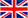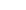English   [Change]

Blog

Categories

Latest Blog

Tags

Research on the Wide Application of Weighing Technology of Combination Weigher

• 2022-01-14

The multihead scale has not been in my country for a long time. With the continuous maturity of technology and the continuous deepening of scientific research, the current multi-head scale has a very broad development prospect in my country, which provides strong support for the development of enterprises.

A typical multihead scale is mainly composed of a feeding tray, a main vibrating plate, a sensor, a collecting hopper and a single-chip microcomputer. The microcontroller is the core component. It can convert the sensor's analog signal into a digital signal, then combine the weights, and then issue control commands. The working steps of the multi-head scale are as follows: feeding → dividing → buffering → weighing → comprehensive calculation → sending instructions → opening the material door → unloading.

The weighing technology of combination weigher applies knowledge of mathematical permutations and combinations. If the multi-head scale has a large number of collection hoppers, m hoppers need to be selected from n collection hoppers. After the combination is determined, select from m collection hoppers. Unload, then re-feed. At the same time, the remaining hoppers must also be combined, the formula is Cm-mm, the previous calculation formula is Cnm. For example, a combination scale has a collection hopper, one collection hopper can weigh 1/a of the total weight, and then the weighing results of all collection hoppers are added to obtain the total weight. The formula for the standard deviation of the total weight is: σ=σ1, where σ1 is the standard deviation of the material and M is the amount of material, equal to the total weight of the material divided by the weight of each material. Using this formula, the deviation is between -2σ and 2σ. Assuming that the weight of each part is 8g, the standard deviation is 0.3g, and the total weight of the material is 240g, the deviation of each part is equal to ×0.3=1.64. The method divides it into 8 parts, the deviation of each part is ×0.3=0.58, and the final deviation is between -1.16 and 1.16.

When testing a multihead scale, the effect of the test conditions on the test results should be considered. Put the weights into the hopper. When weighing, you can change the weight of the weighing piece and observe the change of the data. As a simple example, if the combination scale is unstable during the weighing process, such as shaking, the data will fluctuate within a small range, affecting the accuracy of the results. Let's take a weight of 1000g as an example, put it on the hopper, and observe the weighing indication at different time points. At 8:00, the indication is 999.87, and the error is -0.13; at 9:00, the indication is 999.92, and the error is -0.08; at 10:00, the indication is 1000.07, and the error is +0.07; at 11 o'clock, the indication is 999.95 , the error is -0.05. The results show that the results obtained by this test method meet the accuracy requirements.

To sum up, in the specific application process, the combined weighing technology can be automated, which is convenient for the staff to observe. At the same time, the measurement accuracy is high, which can well meet the needs of society. However, it should be noted that there is still a lot of room for improvement in terms of specific device selection and hardware and software design. It is believed that in the future development, the combination weighing technology can be continuously innovated, and enterprises can manufacture and produce higher quality combination weighing, and promote social progress

Copyright © GUANGDONG GANTAN PACKAGING MACHINERY CO.,LTD |粤公网安备44011302001191号 | 粤ICP备16015233号If you want to get our multi head weighing solutions, please fill your contact info below, so that we can get connection with you.